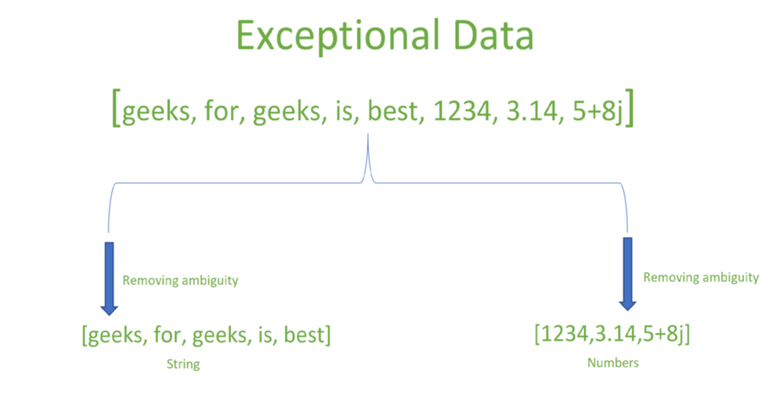Open in App
Not now

# Removing or Modifying the Exceptions during runtime

• Difficulty Level : Expert
• Last Updated : 02 Mar, 2023

Modifying Exceptions During Runtime: Suppose there is a Registration Form. If you got exceptional data in the form like in the place of Name you got phone numbers to remove that ambiguity from the data we use the following logical abstraction without any explicit coding(if-else ladder) not only for registration form can be applied to DBMS Data and also various Datasets.

Exceptional Data: The Data is said to be exceptional when data is incorrect or does not belong to the same group. this data is also called ambiguous data to remove this ambiguity in the data some operations needed to be performed. removing exceptional data from the list reduces errors or exceptions while performing operations.

Example: String operations cannot be done on the entire list, because it also has numerical. If we do so exceptions will be raised. So, to avoid exceptions, partition the data in the array into their respective data type.Diagram for Exceptional data

Convert elements of a list to uppercase.

Input: names[] = [‘geeks’, ‘for’, ‘geeks’, ‘is’, ‘best’, 9876543210, 3.142, 5 + 8j, True], convert strings in the list to uppercase and remove unwanted data.
Output: [‘GEEKS’, ‘FOR’, ‘GEEKS’, ‘IS’, ‘BEST’][9876543210, 3.142, 5 + 8j, True]

Recursive Approach: By removing the exceptional data from the list using exception handling.

Logic Skeleton:

function(args):

try:

#operations
except:

#update/remove exceptional data
#update args

functions(args)
return result

Algorithm:

•  Input: Arr[]
•  Initialize exceptional_data = [], Index = 0.
• Iterate over arr and apply uppercase operation on the data in try block to find exceptional data.

i.e try:

while(index < len(names)):
names[index] = names[index].upper()
index += 1

• If exceptional data found, store it in exceptional_data list and remove that data from list. and again call function with new list and index

i.e except:
exception = names.pop(index)
exceptional_data.append(exception)
case_changer(names, index)

• After looping return arr

Below is the Implementation of the above approach:

## Java

 `import` `java.util.ArrayList;` `import` `java.util.List;`   `public` `class` `Main {` `    ``// List to catch exceptional data.` `    ``public` `static` `List

## Python3

 `# Python Program to remove Exceptional` `# data from a data container`   `# Consider names list needed to have only` `# string data type` `names ``=` `[``'geeks'``, ``'for'``, ``'geeks'``, ``'is'``, ``'best'``, ``9876543210``, ``3.142``, ``5` `+` `8j``, ``True``]`   `# List to catch exceptional data.` `exceptional_data ``=` `[]`   `# Exceptional data Exists i.e int,` `# complex and Boolean.` `print``(``'Exceptional data:'``, names)`   `# Initialize index has value to 0` `index ``=` `0`   `# case_changer converts string to` `# uppercase strings`     `def` `case_changer(names, index):`   `    ``# Iterate over all elements to find` `    ``# exceptional data index.` `    ``try``:` `        ``while``(index < ``len``(names)):`   `            ``# Perform operations on` `            ``# non-exceptional data.` `            ``names[index] ``=` `names[index].upper()` `            ``index ``+``=` `1`   `# After finding exceptional data index` `# try to change or remove it.` `    ``except``:` `        ``exception ``=` `names.pop(index)` `        ``exceptional_data.append(exception)`   `# After removing exceptional data continue` `# operations on non-exceptional by` `# calling the function.` `        ``case_changer(names, index)` `    ``return` `names`     `print``(``'Uppercase data  :'``, case_changer(names, index))` `print``(``"Exceptional data:"``, exceptional_data)`

## C#

 `using` `System;` `using` `System.Collections.Generic;`   `public` `class` `Mainn` `{` `    ``// List to catch exceptional data.` `    ``public` `static` `List<``object``> exceptionalData = ``new` `List<``object``>();`   `    ``// case_changer converts string to uppercase strings` `    ``public` `static` `List<``string``> CaseChanger(List<``object``> names, ``int` `index)` `    ``{` `        ``// List to store only uppercased strings` `        ``List<``string``> newNames = ``new` `List<``string``>();`   `        ``// Iterate over all elements to find exceptional data` `        ``while` `(index < names.Count)` `        ``{` `            ``try` `            ``{` `                ``// Perform operations on non-exceptional data` `                ``string` `upperCased = ((``string``)names[index]).ToUpper();` `                ``newNames.Add(upperCased);` `                ``index++;` `            ``}` `            ``catch` `(InvalidCastException e)` `            ``{` `                ``// Catch exceptional data and remove it from the names list` `                ``exceptionalData.Add(names[index]);` `                ``names.RemoveAt(index);` `                ``continue``;` `            ``}` `        ``}` `        ``return` `newNames;` `    ``}`   `    ``public` `static` `void` `Main(``string``[] args)` `    ``{` `        ``// Consider names list needed to have only string data type` `        ``List<``object``> names = ``new` `List<``object``>(``new` `object``[] { ``"geeks"``, ``"for"``, ``"geeks"``, ``"is"``, ``"best"``, 9876543210L, 3.142, ``true` `});` `        ``Console.WriteLine(``"Exceptional data: "` `+ ``string``.Join(``","``, names));`   `        ``// Call caseChanger method to get uppercased strings and remove exceptional data` `        ``List<``string``> uppercaseData = CaseChanger(names, 0);` `        ``Console.WriteLine(``"Uppercase data  : "` `+ ``string``.Join(``","``, uppercaseData));` `        ``Console.WriteLine(``"Exceptional data: "` `+ ``string``.Join(``","``, exceptionalData));` `    ``}` `}`

Output

```Exceptional data: ['geeks', 'for', 'geeks', 'is', 'best', 9876543210, 3.142, (5+8j), True]
Uppercase data  : ['GEEKS', 'FOR', 'GEEKS', 'IS', 'BEST']
Exceptional data: [9876543210, 3.142, (5+8j), True]```

Time Complexity: O(n), where n is the length of the arr.
Auxiliary Space: O(1).

### Advantages of Modifying the Exceptions during runtime:

• Respective operations on the respective list are done easily without explicit coding.
• Data is partitioned easily.
• Exceptions are handled easily and also reduced.
• The Time complexity is also efficient.
• It also can be used to handle larger data in datasets.

My Personal Notes arrow_drop_up
Related Articles